UNITII Relations and Their Properties Relations Binary relations

• Slides: 81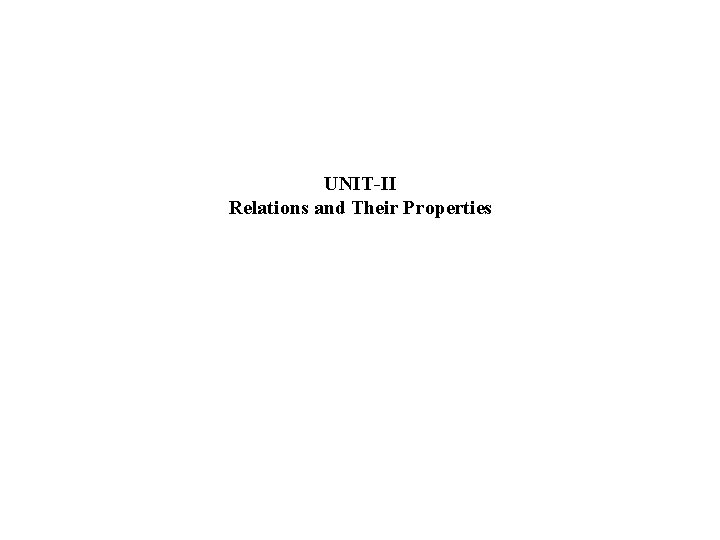UNIT-II Relations and Their Properties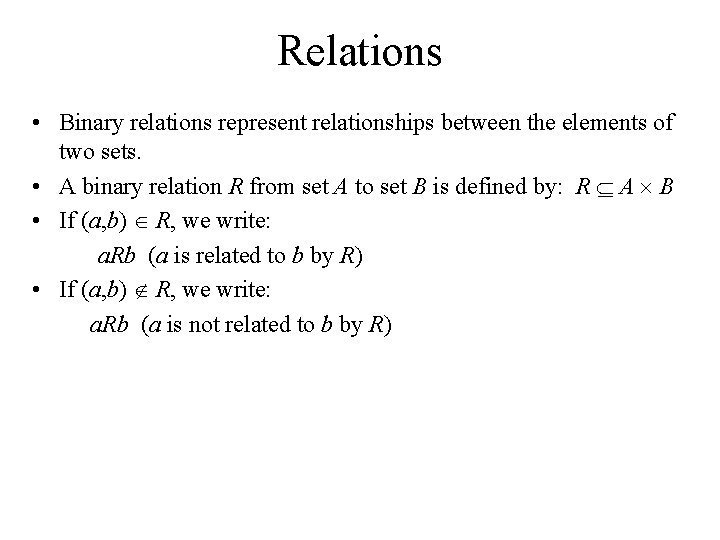Relations • Binary relations represent relationships between the elements of two sets. • A binary relation R from set A to set B is defined by: R A B • If (a, b) R, we write: a. Rb (a is related to b by R) • If (a, b) R, we write: a. Rb (a is not related to b by R)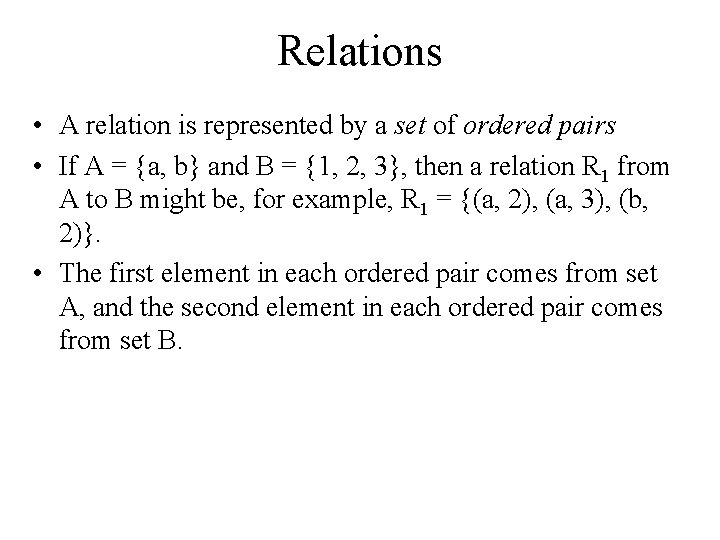Relations • A relation is represented by a set of ordered pairs • If A = {a, b} and B = {1, 2, 3}, then a relation R 1 from A to B might be, for example, R 1 = {(a, 2), (a, 3), (b, 2)}. • The first element in each ordered pair comes from set A, and the second element in each ordered pair comes from set B.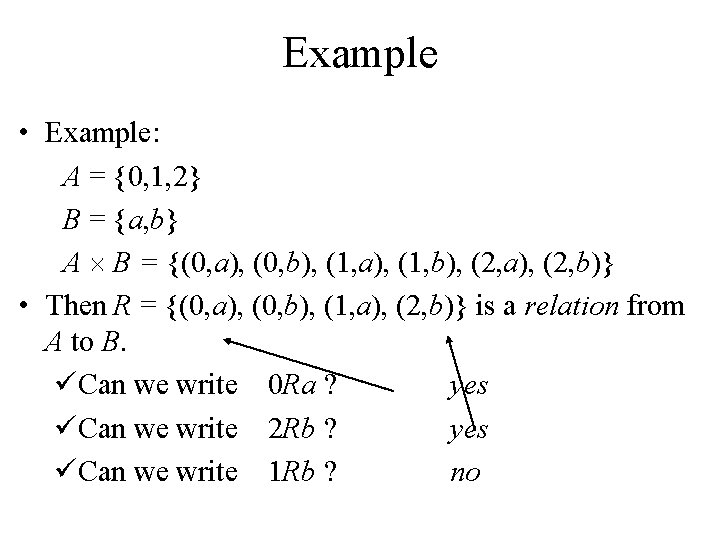Example • Example: A = {0, 1, 2} B = {a, b} A B = {(0, a), (0, b), (1, a), (1, b), (2, a), (2, b)} • Then R = {(0, a), (0, b), (1, a), (2, b)} is a relation from A to B. üCan we write 0 Ra ? yes üCan we write 2 Rb ? yes üCan we write 1 Rb ? noExample • A relation may be represented graphically or as a table: 0 1 a b 2 R a b 0 X X 1 X 2 We can see that 0 Ra but 1 Rb. / XFunctions as Relations • A function is a relation that has the restriction that each element of A can be related to exactly one element of B. Relation 1 Function a b 1 a bRelations on a Set • Relations can also be from a set to itself. • A relation on the set A is a relation from set A to set A, i. e. , R A A • Let A = {1, 2, 3, 4} • Which ordered pairs are in the relation R = {(a, b) | a divides b}? • R = {(1, 1), (1, 2), (1, 3), (1, 4), (2, 2), (2, 4), (3, 3), (4, 4)}Relations on a Set • Which of these relations (on the set of integers) contain each of the pairs (1, 1), (1, 2), (2, 1), (1, -1), and (2, 2)? R 1 = {(a, b) | a b} R 2 = {(a, b) | a > b} R 3 = {(a, b) | a = b, a = b} R 4 = {(a, b) | a = b} R 5 = {(a, b) | a = b + 1} R 6 = {(a, b) | a + b 3}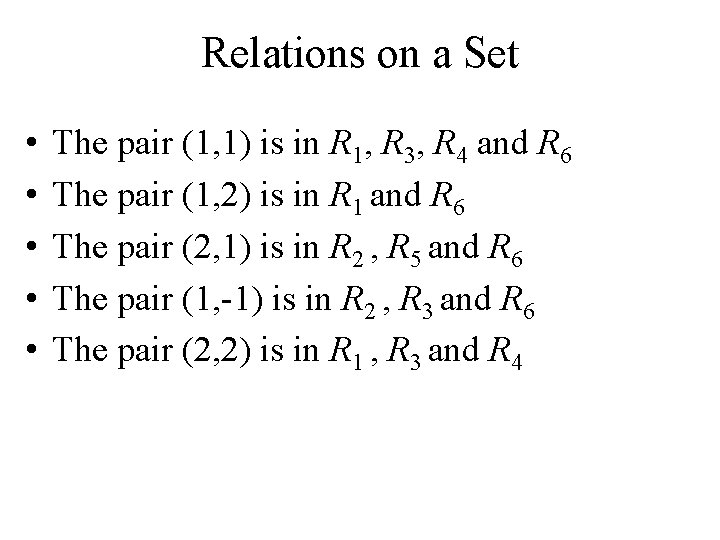Relations on a Set • • • The pair (1, 1) is in R 1, R 3, R 4 and R 6 The pair (1, 2) is in R 1 and R 6 The pair (2, 1) is in R 2 , R 5 and R 6 The pair (1, -1) is in R 2 , R 3 and R 6 The pair (2, 2) is in R 1 , R 3 and R 4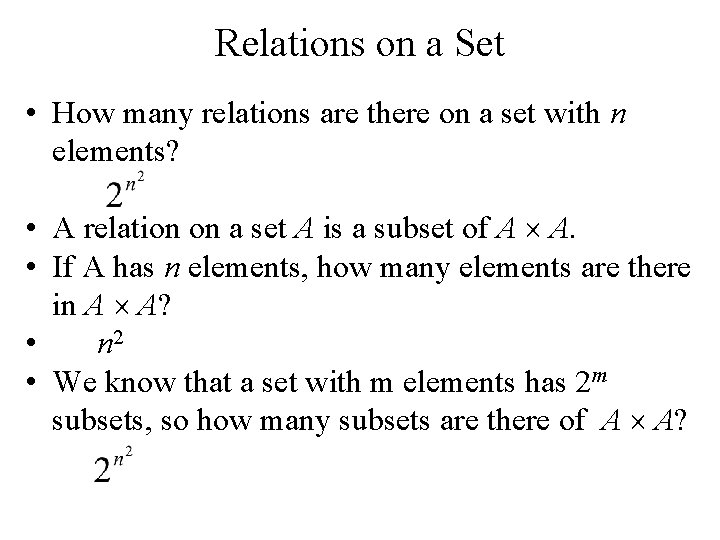Relations on a Set • How many relations are there on a set with n elements? • A relation on a set A is a subset of A A. • If A has n elements, how many elements are there in A A? • n 2 • We know that a set with m elements has 2 m subsets, so how many subsets are there of A A?Relations on a Set • There are relations on a set with n elements. • How many relations are there on set S = {a, b, c}? • There are 3 elements in set S, so S S has 32 = 9 elements. • Therefore, there are 29 = 512 different relations on the set S = {a, b, c}.Properties of Relations • Let R be a relation on set A. • R is reflexive if: (a, a) R for every element a A.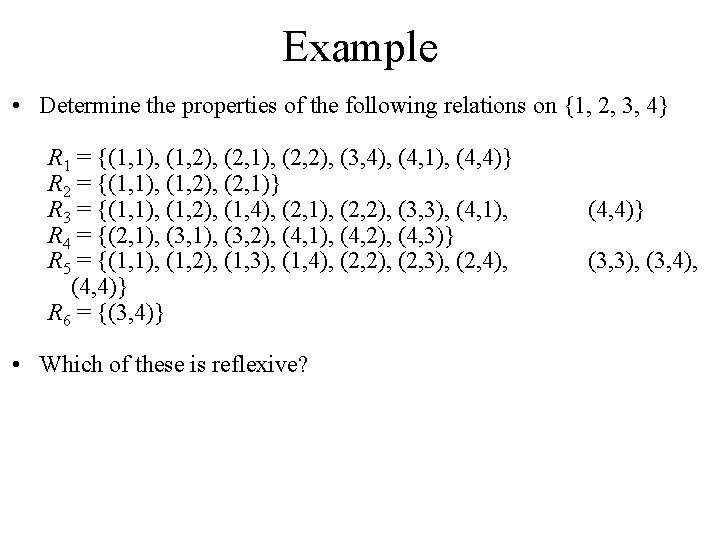Example • Determine the properties of the following relations on {1, 2, 3, 4} R 1 = {(1, 1), (1, 2), (2, 1), (2, 2), (3, 4), (4, 1), (4, 4)} R 2 = {(1, 1), (1, 2), (2, 1)} R 3 = {(1, 1), (1, 2), (1, 4), (2, 1), (2, 2), (3, 3), (4, 1), R 4 = {(2, 1), (3, 2), (4, 1), (4, 2), (4, 3)} R 5 = {(1, 1), (1, 2), (1, 3), (1, 4), (2, 2), (2, 3), (2, 4), (4, 4)} R 6 = {(3, 4)} • Which of these is reflexive? (4, 4)} (3, 3), (3, 4),Example • Which of these is reflexive? R 1 = {(1, 1), (1, 2), (2, 1), (2, 2), (3, 4), (4, 1), (4, 4)} R 2 = {(1, 1), (1, 2), (2, 1)} R 3 = {(1, 1), (1, 2), (1, 4), (2, 1), (2, 2), (3, 3), (4, 1), (4, 4)} R 4 = {(2, 1), (3, 2), (4, 1), (4, 2), (4, 3)} R 5 = {(1, 1), (1, 2), (1, 3), (1, 4), (2, 2), (2, 3), (2, 4), (3, 3), (3, 4), (4, 4)} R 6 = {(3, 4)} • The relations R 3 and R 5 are reflexive because they contain all pairs of the form (a, a); the other don’t [they are all missing (3, 3)].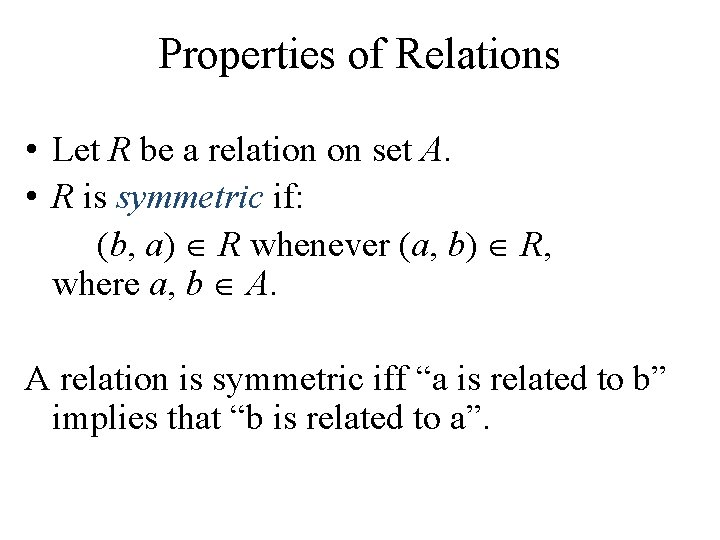Properties of Relations • Let R be a relation on set A. • R is symmetric if: (b, a) R whenever (a, b) R, where a, b A. A relation is symmetric iff “a is related to b” implies that “b is related to a”.Example • Which of these is symmetric? R 1 = {(1, 1), (1, 2), (2, 1), (2, 2), (3, 4), (4, 1), (4, 4)} R 2 = {(1, 1), (1, 2), (2, 1)} R 3 = {(1, 1), (1, 2), (1, 4), (2, 1), (2, 2), (3, 3), (4, 1), (4, 4)} R 4 = {(2, 1), (3, 2), (4, 1), (4, 2), (4, 3)} R 5 = {(1, 1), (1, 2), (1, 3), (1, 4), (2, 2), (2, 3), (2, 4), (3, 3), (3, 4), (4, 4)} R 6 = {(3, 4)} • The relations R 2 and R 3 are symmetric because in each case (b, a) belongs to the relation whenever (a, b) does. • The other relations aren’t symmetric.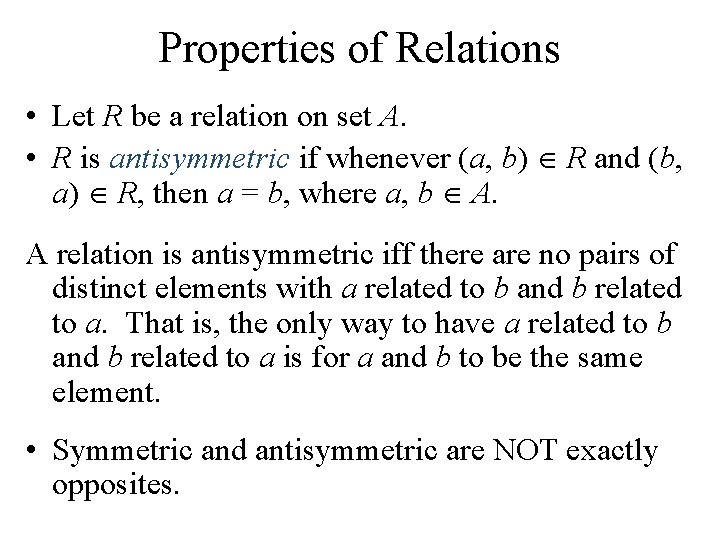Properties of Relations • Let R be a relation on set A. • R is antisymmetric if whenever (a, b) R and (b, a) R, then a = b, where a, b A. A relation is antisymmetric iff there are no pairs of distinct elements with a related to b and b related to a. That is, the only way to have a related to b and b related to a is for a and b to be the same element. • Symmetric and antisymmetric are NOT exactly opposites.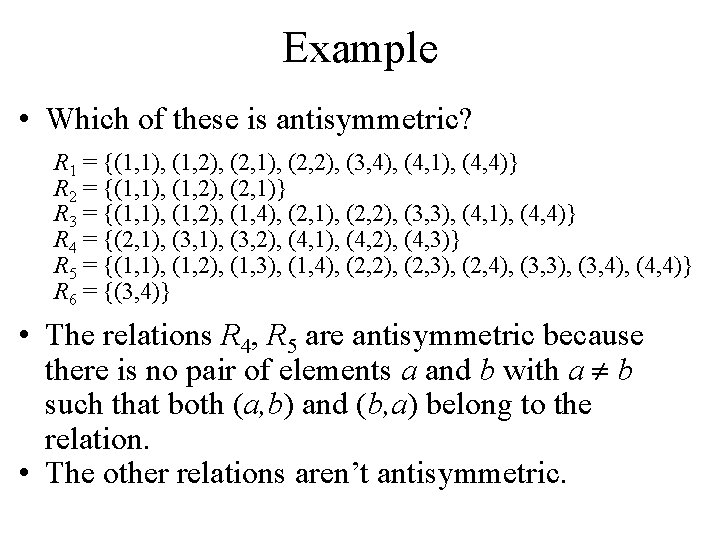Example • Which of these is antisymmetric? R 1 = {(1, 1), (1, 2), (2, 1), (2, 2), (3, 4), (4, 1), (4, 4)} R 2 = {(1, 1), (1, 2), (2, 1)} R 3 = {(1, 1), (1, 2), (1, 4), (2, 1), (2, 2), (3, 3), (4, 1), (4, 4)} R 4 = {(2, 1), (3, 2), (4, 1), (4, 2), (4, 3)} R 5 = {(1, 1), (1, 2), (1, 3), (1, 4), (2, 2), (2, 3), (2, 4), (3, 3), (3, 4), (4, 4)} R 6 = {(3, 4)} • The relations R 4, R 5 are antisymmetric because there is no pair of elements a and b with a b such that both (a, b) and (b, a) belong to the relation. • The other relations aren’t antisymmetric.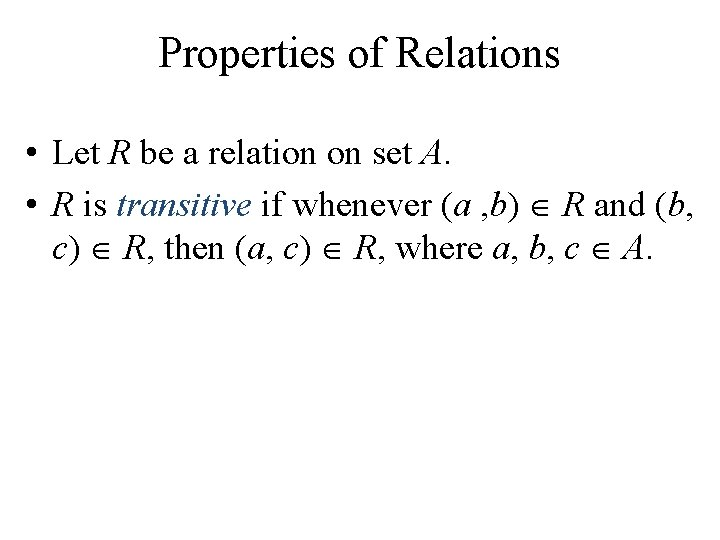Properties of Relations • Let R be a relation on set A. • R is transitive if whenever (a , b) R and (b, c) R, then (a, c) R, where a, b, c A.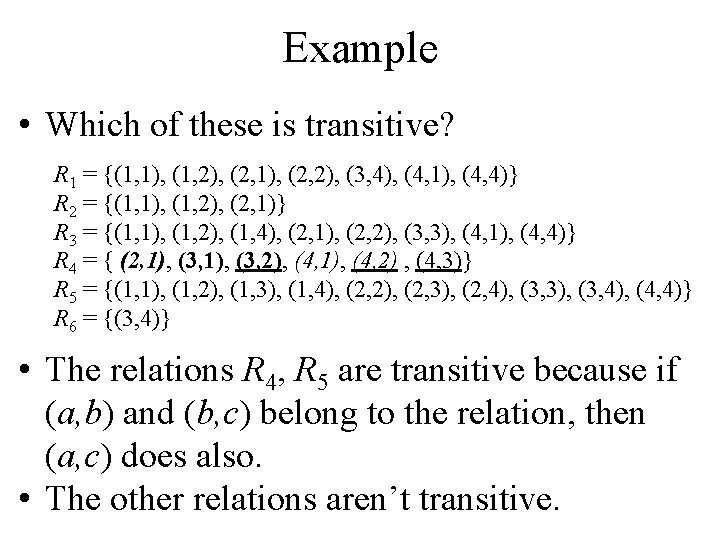Example • Which of these is transitive? R 1 = {(1, 1), (1, 2), (2, 1), (2, 2), (3, 4), (4, 1), (4, 4)} R 2 = {(1, 1), (1, 2), (2, 1)} R 3 = {(1, 1), (1, 2), (1, 4), (2, 1), (2, 2), (3, 3), (4, 1), (4, 4)} R 4 = { (2, 1), (3, 2), (4, 1), (4, 2) , (4, 3)} R 5 = {(1, 1), (1, 2), (1, 3), (1, 4), (2, 2), (2, 3), (2, 4), (3, 3), (3, 4), (4, 4)} R 6 = {(3, 4)} • The relations R 4, R 5 are transitive because if (a, b) and (b, c) belong to the relation, then (a, c) does also. • The other relations aren’t transitive.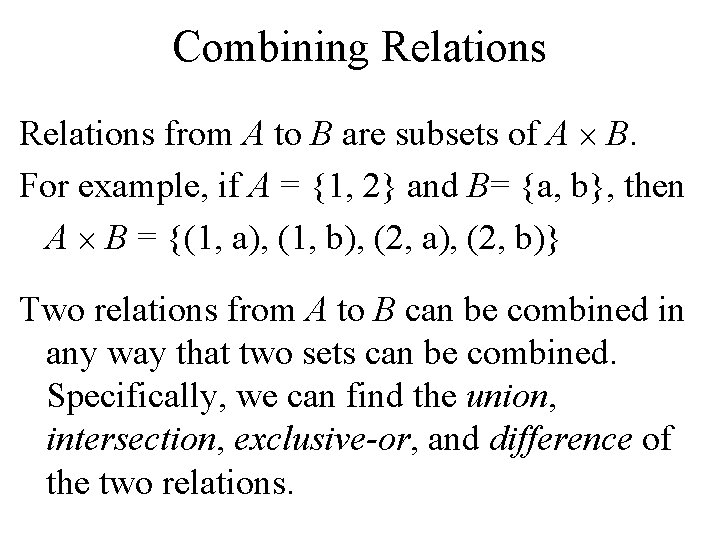Combining Relations from A to B are subsets of A B. For example, if A = {1, 2} and B= {a, b}, then A B = {(1, a), (1, b), (2, a), (2, b)} Two relations from A to B can be combined in any way that two sets can be combined. Specifically, we can find the union, intersection, exclusive-or, and difference of the two relations.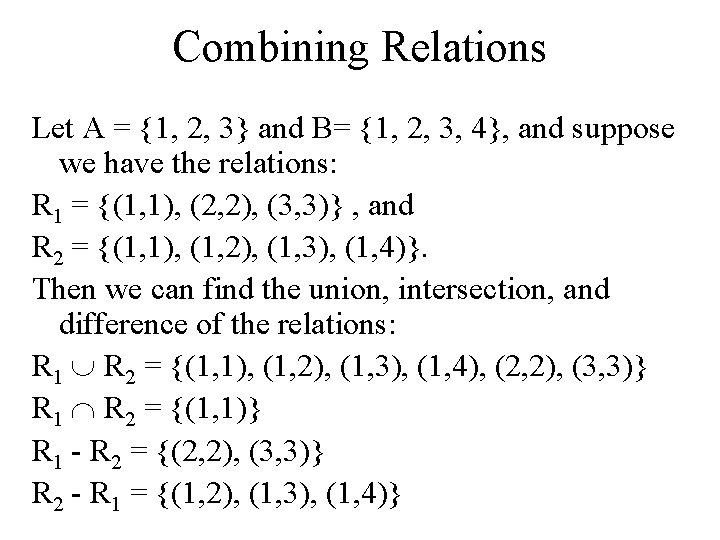Combining Relations Let A = {1, 2, 3} and B= {1, 2, 3, 4}, and suppose we have the relations: R 1 = {(1, 1), (2, 2), (3, 3)} , and R 2 = {(1, 1), (1, 2), (1, 3), (1, 4)}. Then we can find the union, intersection, and difference of the relations: R 1 R 2 = {(1, 1), (1, 2), (1, 3), (1, 4), (2, 2), (3, 3)} R 1 R 2 = {(1, 1)} R 1 - R 2 = {(2, 2), (3, 3)} R 2 - R 1 = {(1, 2), (1, 3), (1, 4)}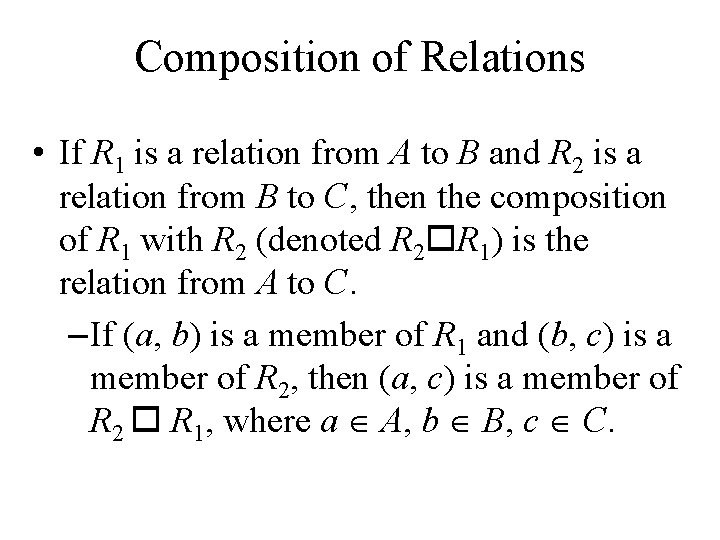Composition of Relations • If R 1 is a relation from A to B and R 2 is a relation from B to C, then the composition of R 1 with R 2 (denoted R 2 R 1) is the relation from A to C. – If (a, b) is a member of R 1 and (b, c) is a member of R 2, then (a, c) is a member of R 2 R 1, where a A, b B, c C.Example • Let A={a, b, c}, B={w, x, y, z}, C={A, B, C, D} R 1={(a, z), (b, w)}, R 2={(w, B), (w, D), (x, A)} • Find R 2 R 1 • Match (b, c) with (a, b) to get (a, c) • R 2’s b’s are w and x; R 1’s b’s are z and w • Only the w’s match; R 1 has only 1 w pair, (b, w) • So the (a, c) pairs will include b from R 1 and B and D from R 2: (b, B), (b, D)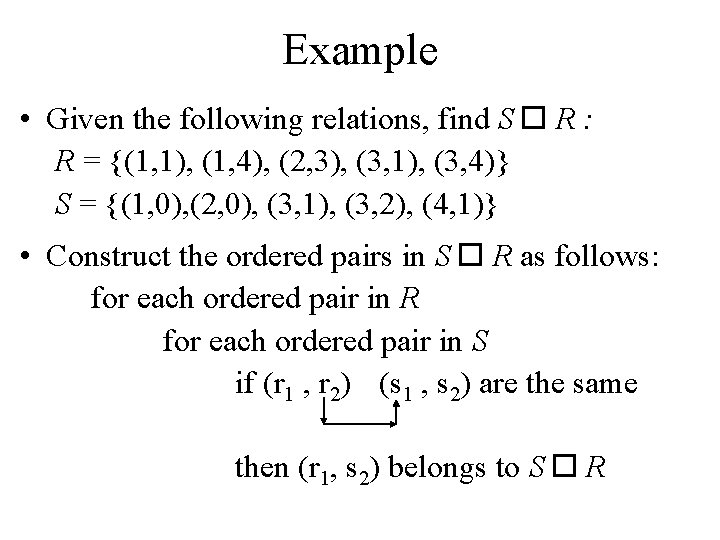Example • Given the following relations, find S R : R = {(1, 1), (1, 4), (2, 3), (3, 1), (3, 4)} S = {(1, 0), (2, 0), (3, 1), (3, 2), (4, 1)} • Construct the ordered pairs in S R as follows: for each ordered pair in R for each ordered pair in S if (r 1 , r 2) (s 1 , s 2) are the same then (r 1, s 2) belongs to S R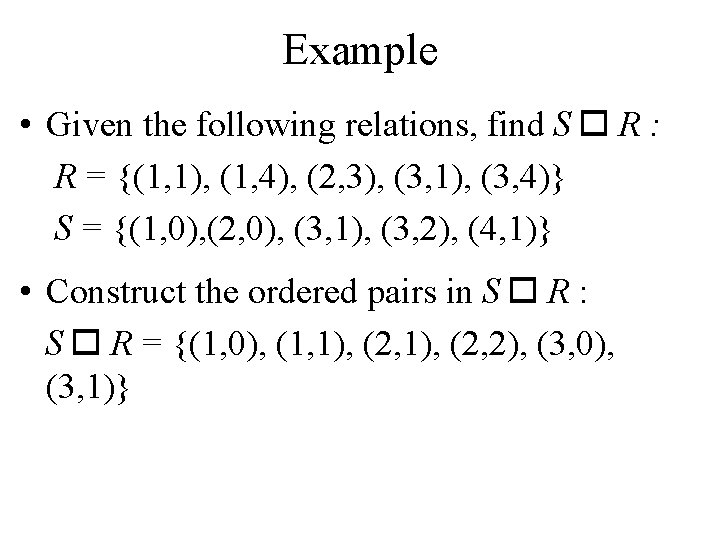Example • Given the following relations, find S R : R = {(1, 1), (1, 4), (2, 3), (3, 1), (3, 4)} S = {(1, 0), (2, 0), (3, 1), (3, 2), (4, 1)} • Construct the ordered pairs in S R : S R = {(1, 0), (1, 1), (2, 2), (3, 0), (3, 1)}The Powers of a Relation • The powers of a relation R are recursively defined from the definition of a composite of two relations. • Let R be a relation on the set A. The powers Rn, for n = 1, 2, 3, … are defined recursively by: R 1 = R Rn+1 = Rn R : So: R 2 = R R R 3 = R 2 R = (R R) etc.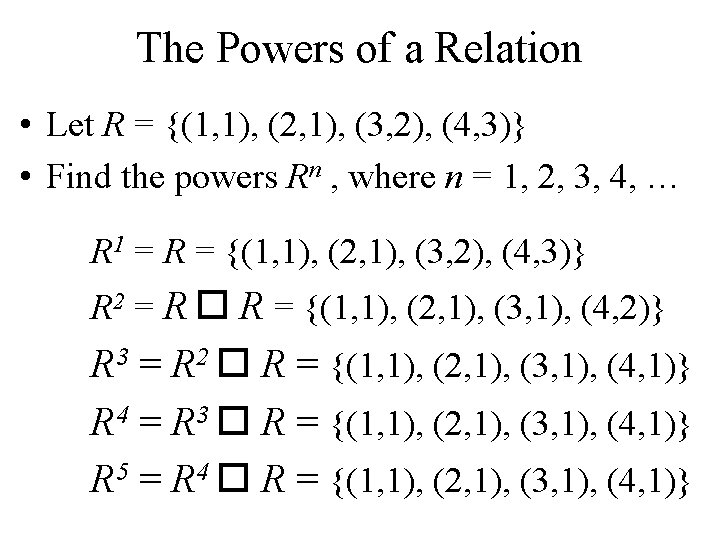The Powers of a Relation • Let R = {(1, 1), (2, 1), (3, 2), (4, 3)} • Find the powers Rn , where n = 1, 2, 3, 4, … R 1 = R = {(1, 1), (2, 1), (3, 2), (4, 3)} R 2 = R R = {(1, 1), (2, 1), (3, 1), (4, 2)} R 3 = R 2 R = {(1, 1), (2, 1), (3, 1), (4, 1)} R 4 = R 3 R = {(1, 1), (2, 1), (3, 1), (4, 1)} R 5 = R 4 R = {(1, 1), (2, 1), (3, 1), (4, 1)}The Powers of a Relation • The relation R on a set A is transitive iff Rn R for n = 1, 2, 3, 4, …Representing RelationsRepresenting Relations Using Matrices • Let R be a relation from A to B A = {a 1, a 2, … , am} B = {b 1, b 2, … , bn} • The zero-one matrix representing the relation R has a 1 as its (i, j) entry when ai is related to bj and a 0 in this position if ai is not related to bj.Example • Let R be a relation from A to B A={a, b, c} B={d, e} R={(a, d), (b, e), (c, d)} • Find the relation matrix for RRelation Matrix Let R be a relation from A to B A={a, b, c} B={d, e} R={(a, d), (b, e), (c, d)} Note that A is represented by the rows and B by the columns in the matrix. Cellij in the matrix contains a 1 iff ai is related to bj.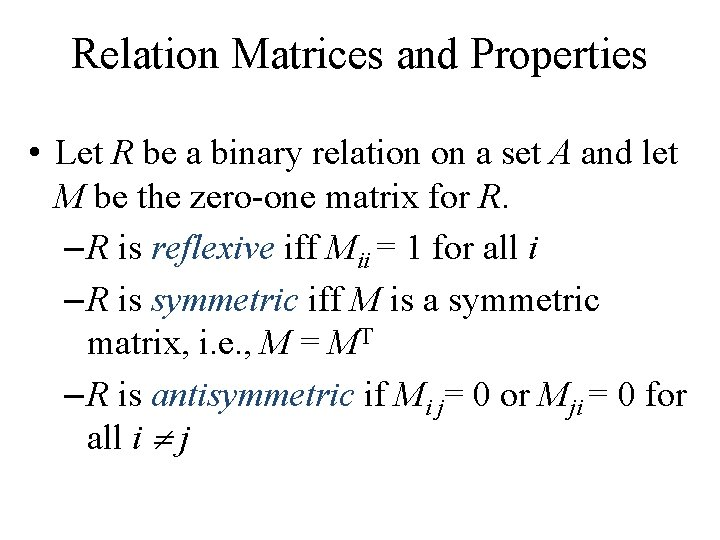Relation Matrices and Properties • Let R be a binary relation on a set A and let M be the zero-one matrix for R. – R is reflexive iff Mii = 1 for all i – R is symmetric iff M is a symmetric matrix, i. e. , M = MT – R is antisymmetric if Mi j= 0 or Mji = 0 for all i j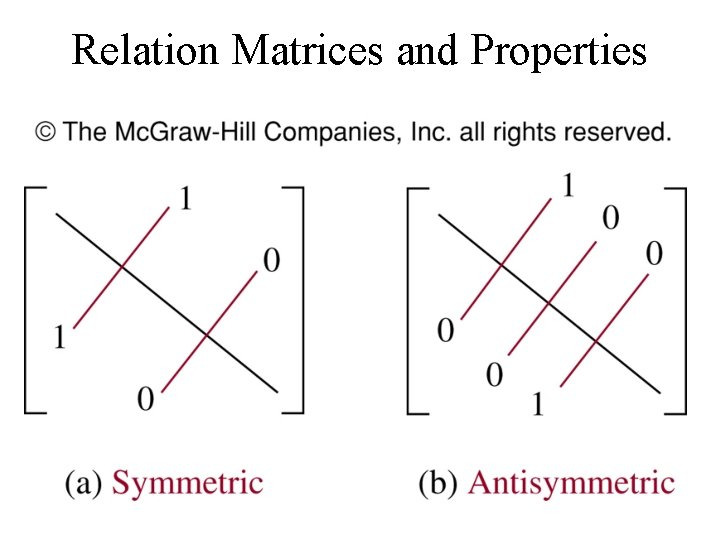Relation Matrices and PropertiesExample • Suppose that the relation R on a set is represented by the matrix MR. é 1 1 0ù ê ú M R = ê 1 1 1ú êë 0 1 1úû • Is R reflexive, symmetric, and / or antisymmetric?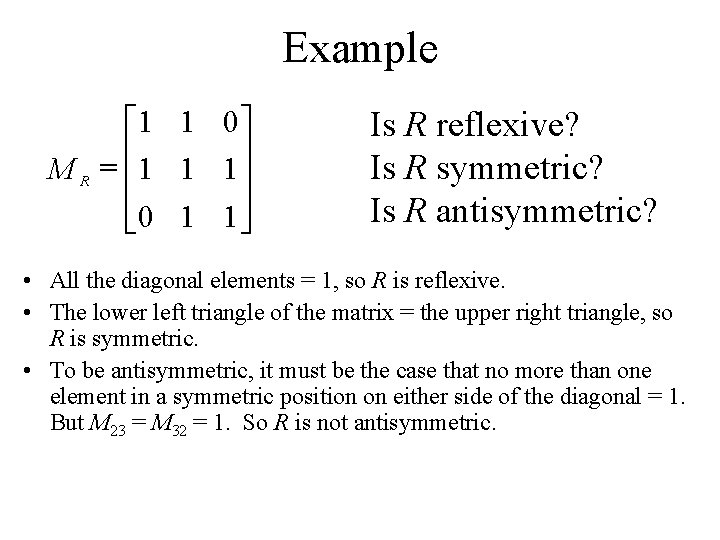Example é 1 1 0ù M R = êê 1 1 1úú êë 0 1 1úû Is R reflexive? Is R symmetric? Is R antisymmetric? • All the diagonal elements = 1, so R is reflexive. • The lower left triangle of the matrix = the upper right triangle, so R is symmetric. • To be antisymmetric, it must be the case that no more than one element in a symmetric position on either side of the diagonal = 1. But M 23 = M 32 = 1. So R is not antisymmetric.Representing Relations Using Digraphs • Represent: – each element of the set by a point – each ordered pair using an arc with its direction indicated by an arrowRepresenting Relations Using Digraphs • A directed graph (or digraph) consists of a set V of vertices (or nodes) together with a set E of ordered pairs of elements of V called edges (or arcs). • The vertex a is called the initial vertex of the edge (a, b). • The vertex b is called the terminal vertex of the edge (a, b).Example • Let R be a relation on set A A={a, b, c} R={(a, b), (a, c), (b, b), (c, a), (c, b)}. • Draw the digraph that represents R a b c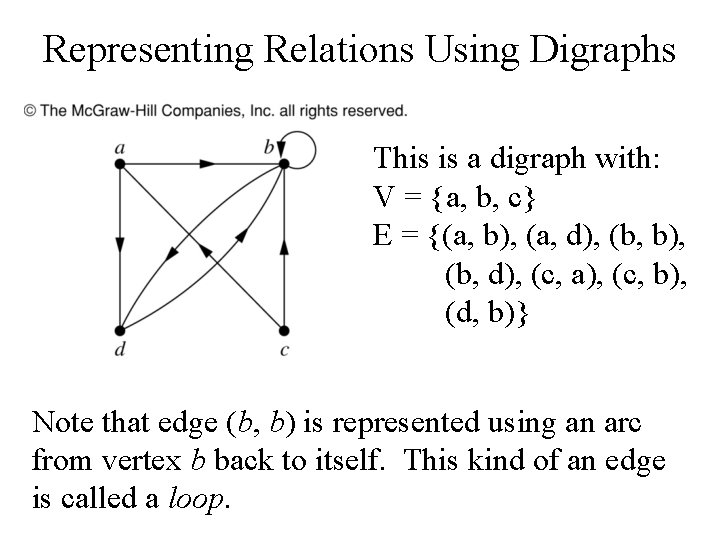Representing Relations Using Digraphs This is a digraph with: V = {a, b, c} E = {(a, b), (a, d), (b, b), (b, d), (c, a), (c, b), (d, b)} Note that edge (b, b) is represented using an arc from vertex b back to itself. This kind of an edge is called a loop.Example What are the ordered pairs in the relation R represented by the directed graph to the left? This digraph represents the relation R = {(1, 1), (1, 3), (2, 1), (2, 3), (2, 4), (3, 1), on the set {1, 2, 3, 4}. (3, 2), (4, 1)}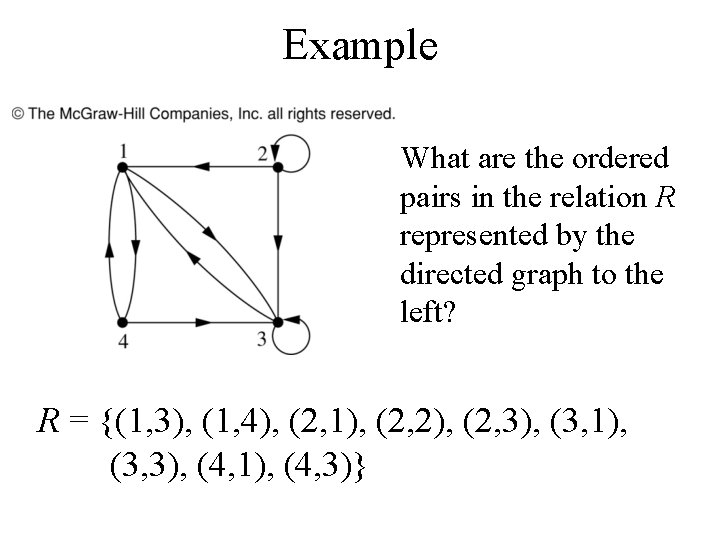Example What are the ordered pairs in the relation R represented by the directed graph to the left? R = {(1, 3), (1, 4), (2, 1), (2, 2), (2, 3), (3, 1), (3, 3), (4, 1), (4, 3)}Example According to the digraph representing R: • is (4, 3) an ordered pair in R? • is (3, 4) an ordered pair in R? • is (3, 3) an ordered pair in R? (4, 3) is an ordered pair in R (3, 4) is not an ordered pair in R – no arrowhead pointing from 3 to 4 (3, 3) is an ordered pair in R – loop back to itself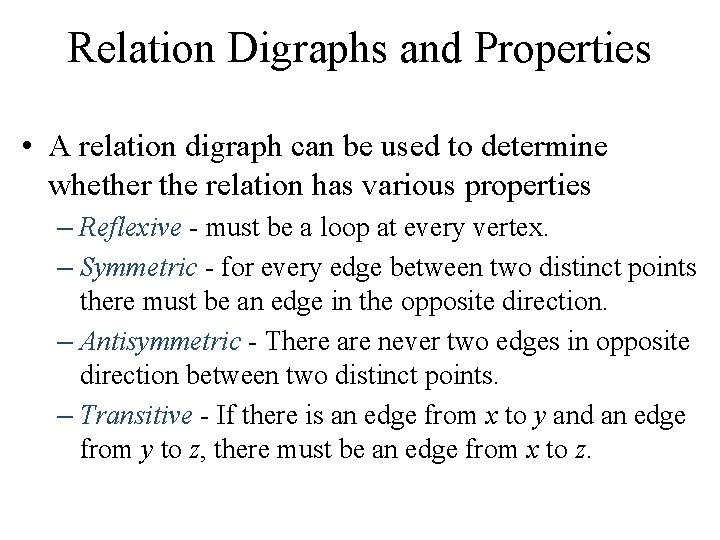Relation Digraphs and Properties • A relation digraph can be used to determine whether the relation has various properties – Reflexive - must be a loop at every vertex. – Symmetric - for every edge between two distinct points there must be an edge in the opposite direction. – Antisymmetric - There are never two edges in opposite direction between two distinct points. – Transitive - If there is an edge from x to y and an edge from y to z, there must be an edge from x to z.Example According to the digraph representing R: • is R reflexive? • is R symmetric? • is R antisymmetric? • is R transitive? • R is reflexive – there is a loop at every vertex • R is not symmetric – there is an edge from a to b but not from b to a • R is not antisymmetric – there are edges in both directions connecting b and c • R is not transitive – there is an edge from a to b and an edge from b to c, but not from a to cExample According to the digraph representing S: • is S reflexive? • is S symmetric? • is S antisymmetric? • is S transitive? • S is not reflexive – there aren’t loops at every vertex • S is symmetric – for every edge from one distinct vertex to another, there is a matching edge in the opposite direction • S is not antisymmetric – there are edges in both directions connecting a and b • S is not transitive – there is an edge from c to a and an edge from a to b, but not from c to b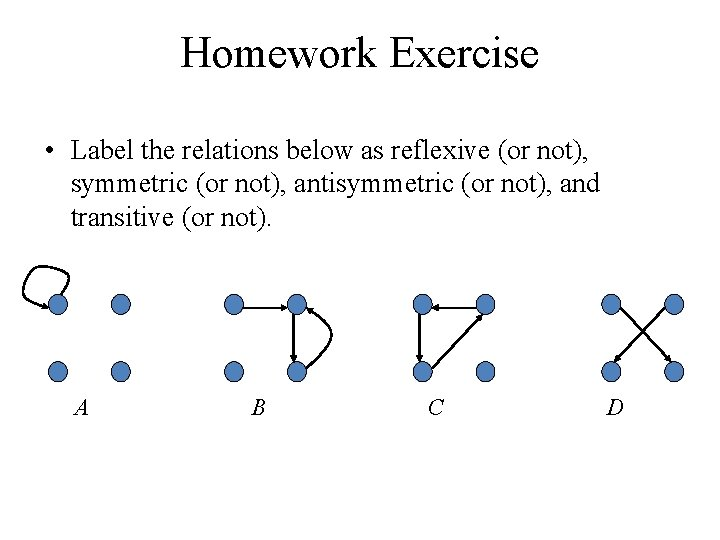Homework Exercise • Label the relations below as reflexive (or not), symmetric (or not), antisymmetric (or not), and transitive (or not). A B C DEquivalence Relations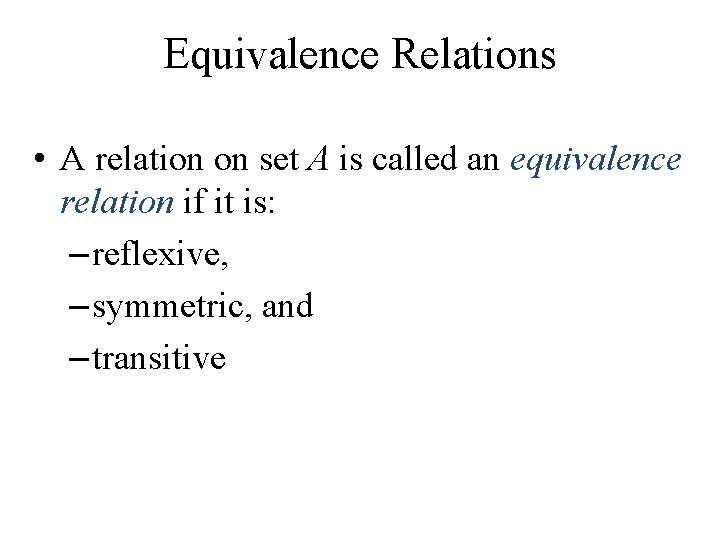Equivalence Relations • A relation on set A is called an equivalence relation if it is: – reflexive, – symmetric, and – transitive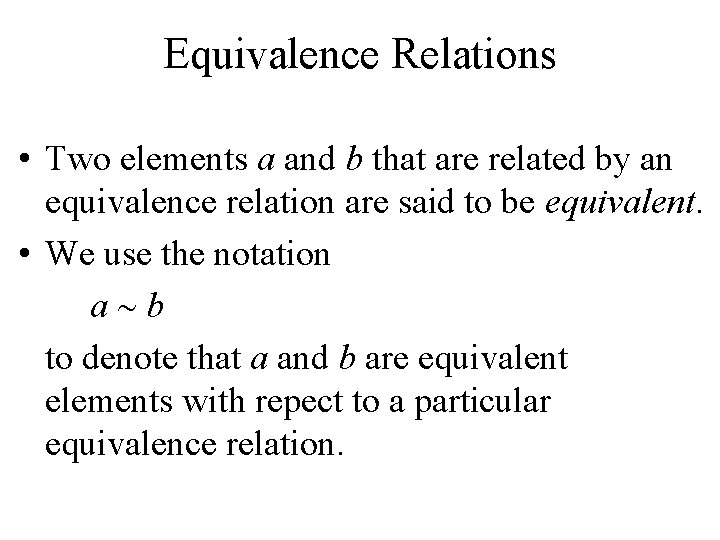Equivalence Relations • Two elements a and b that are related by an equivalence relation are said to be equivalent. • We use the notation a b to denote that a and b are equivalent elements with repect to a particular equivalence relation.Example • Let R be a relation on set A, where A = {1, 2, 3, 4, 5} and R = {(1, 1), (2, 2), (3, 3), (4, 4), (5, 5), (1, 3), (3, 1)} • Is R an equivalence relation? • We can solve this by drawing a relation digraph: – Reflexive – there must be a loop at every vertex. – Symmetric - for every edge between two distinct points there must be an edge in the opposite direction. – Transitive - if there is an edge from x to y and an edge from y to z, there must be an edge from x to z.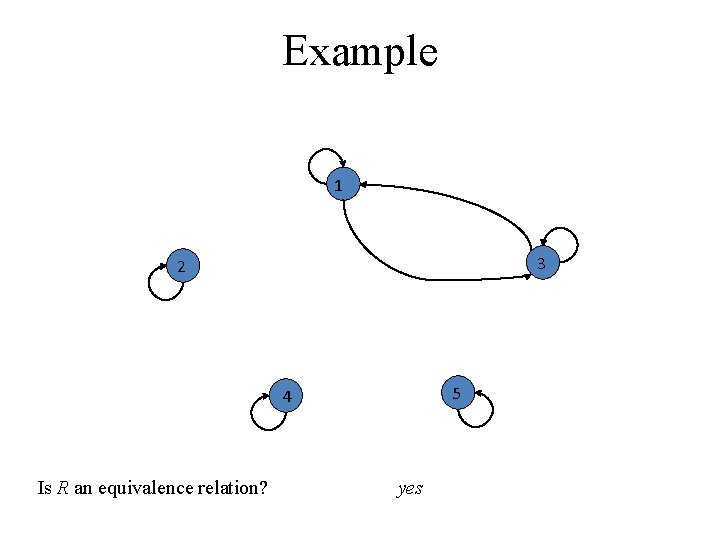Example 1 3 2 5 4 Is R an equivalence relation? yesExample – Congruence modulo m • Let R = {(a, b) | a b (mod m)} be a relation on the set of integers and m be a positive integer > 1. Is R an equivalence relation? – Reflexive – is it true that a a (mod m)}? yes – Symmetric – is it true that if a b (mod m) then b a (mod m)? yes – Transitive - is it true that if a b (mod m) and b c (mod m) then a c (mod m)? yesExample • Suppose that R is the relation on the set of strings of English letters such that a. Rb iff l(a) = l(b), where l(x) is the length of the string x. • Is R an equivalence relation?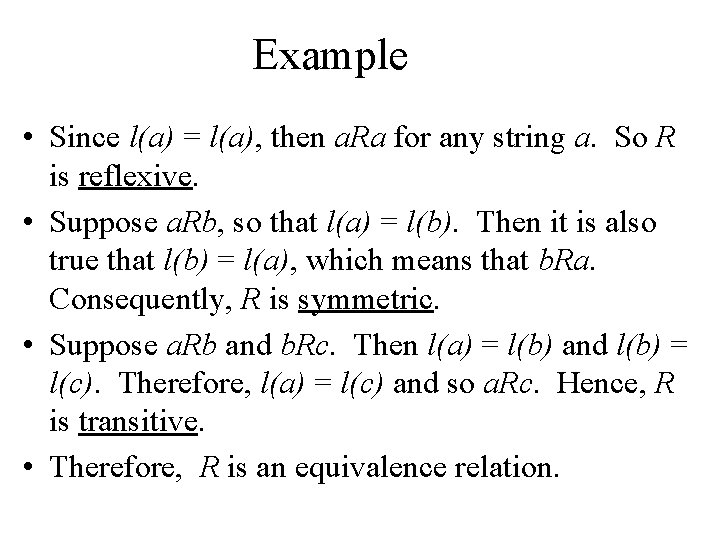Example • Since l(a) = l(a), then a. Ra for any string a. So R is reflexive. • Suppose a. Rb, so that l(a) = l(b). Then it is also true that l(b) = l(a), which means that b. Ra. Consequently, R is symmetric. • Suppose a. Rb and b. Rc. Then l(a) = l(b) and l(b) = l(c). Therefore, l(a) = l(c) and so a. Rc. Hence, R is transitive. • Therefore, R is an equivalence relation.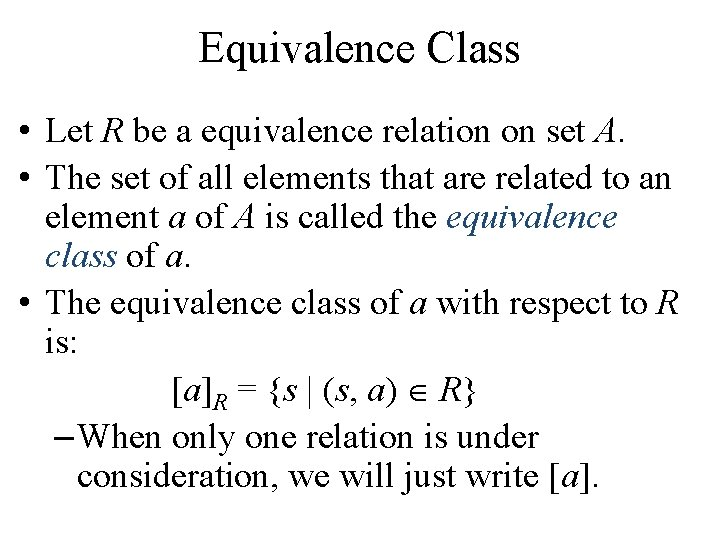Equivalence Class • Let R be a equivalence relation on set A. • The set of all elements that are related to an element a of A is called the equivalence class of a. • The equivalence class of a with respect to R is: [a]R = {s | (s, a) R} – When only one relation is under consideration, we will just write [a].Equivalence Class • If R is a equivalence relation on a set A, the equivalence class of the element a is: [a]R = {s | (s, a) R} If b [a]R , then b is called a representative of this equivalence class.Equivalence Class • Let R be the relation on the set of integers such that a. Rb iff a = b or a = -b. We can show that this is an equivalence relation. • The equivalence class of element a is [a] = {a, -a} • Examples:  = {7, -7}  = {0} [-5] = {5, -5}Equivalence Example • Consider the equivalence relation R on set A. What are the equivalence classes? A = {1, 2, 3, 4, 5} R = {(1, 1), (2, 2), (3, 3), (4, 4), (5, 5), (1, 3), (3, 1)} • Just look at the a. Rb relationships. Which elements are related to which?  = {1, 3}  = {2}  = {3, 1}  = {4}  = {5}A useful theorem about classes • Let R be an equivalence relation on a set A. These statements for a and b of A are equivalent: a. Rb [a] = [b] [a] [b]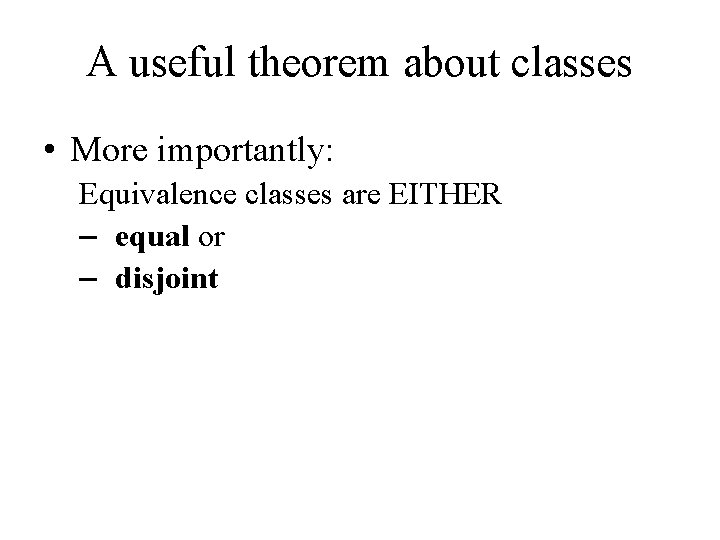A useful theorem about classes • More importantly: Equivalence classes are EITHER – equal or – disjointPartitions • A partition of a set A divides A into nonoverlapping subsets: – A partition of a set A is a collection of disjoint nonempty subsets of A that have A as their union. Example 1 A 2 A 1 A 6 A 3 A 5 A 4 Set APartitions • A partition of a set A divides A into non-overlapping subsets: • Let S be a given set and A={A 1, A 2, …. Am} where each Ai, i 1, 2… is a subset of S • Set A is called covering of S and sets. A 1, A 2, … are said to cover S – A partition of a set A is a collection of disjoint nonempty subsets of A that have A as their union. Then the subsets are called blocks of the partition. • Example 2 S = {a, b, c, d, e, f } S 1 = {a, d, e} S 2 = {b} S 3 = {c, f } P = {S 1, S 2, S 3} P is a partition of set SPartitions and Equivalence Relations • Let R be an equivalence relation on set S – then the equivalence classes of R form a partition of S • Conversely, if {Ai | i I } is a partition of set S, – then there is an equivalence relation R that has the sets Ai (i I) as its equivalence classes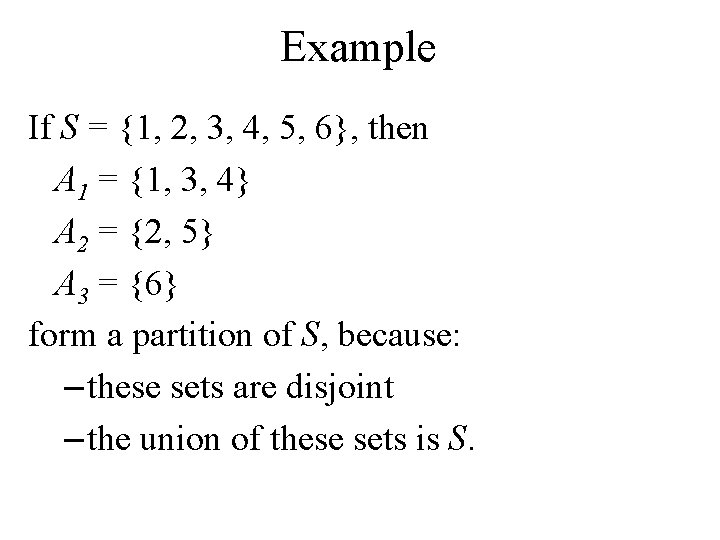Example If S = {1, 2, 3, 4, 5, 6}, then A 1 = {1, 3, 4} A 2 = {2, 5} A 3 = {6} form a partition of S, because: – these sets are disjoint – the union of these sets is S.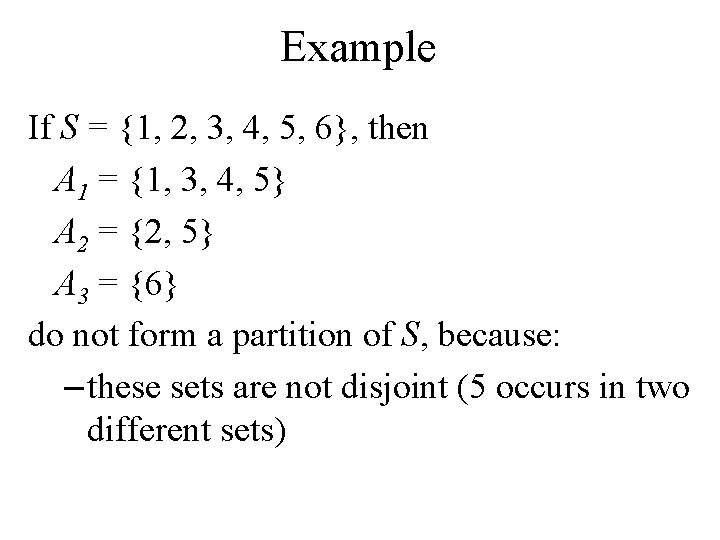Example If S = {1, 2, 3, 4, 5, 6}, then A 1 = {1, 3, 4, 5} A 2 = {2, 5} A 3 = {6} do not form a partition of S, because: – these sets are not disjoint (5 occurs in two different sets)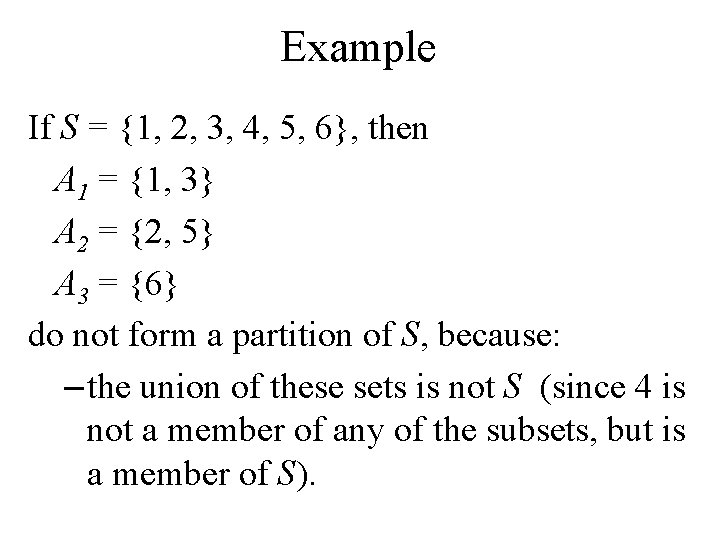Example If S = {1, 2, 3, 4, 5, 6}, then A 1 = {1, 3} A 2 = {2, 5} A 3 = {6} do not form a partition of S, because: – the union of these sets is not S (since 4 is not a member of any of the subsets, but is a member of S).Example If S = {1, 2, 3, 4, 5, 6}, then A 1 = {1, 3, 4} A 2 = {2, 5} A 3 = {6, 7} do not form a partition of S, because: – the union of these sets is not S (since 7 is a member of set A 3 but is not a member of S).Constructing an Equivalence Relation from a Partition Given set S = {1, 2, 3, 4, 5, 6} and a partition of S, A 1 = {1, 2, 3} A 2 = {4, 5} A 3 = {6} then we can find the ordered pairs that make up the equivalence relation R produced by that partition.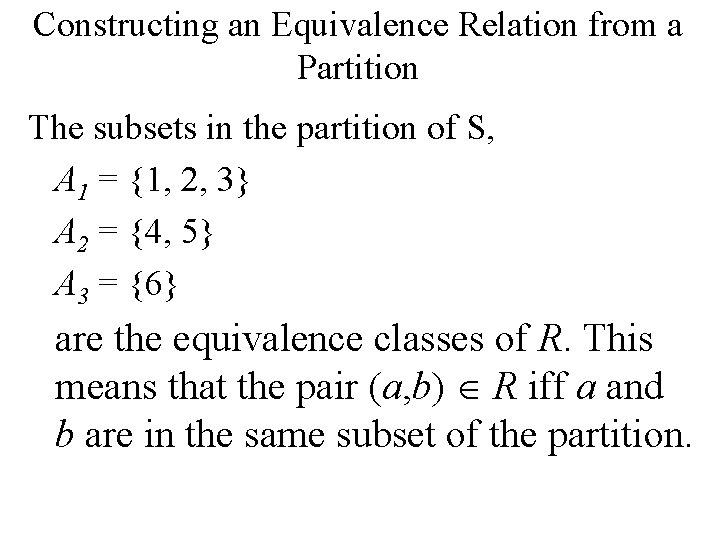Constructing an Equivalence Relation from a Partition The subsets in the partition of S, A 1 = {1, 2, 3} A 2 = {4, 5} A 3 = {6} are the equivalence classes of R. This means that the pair (a, b) R iff a and b are in the same subset of the partition.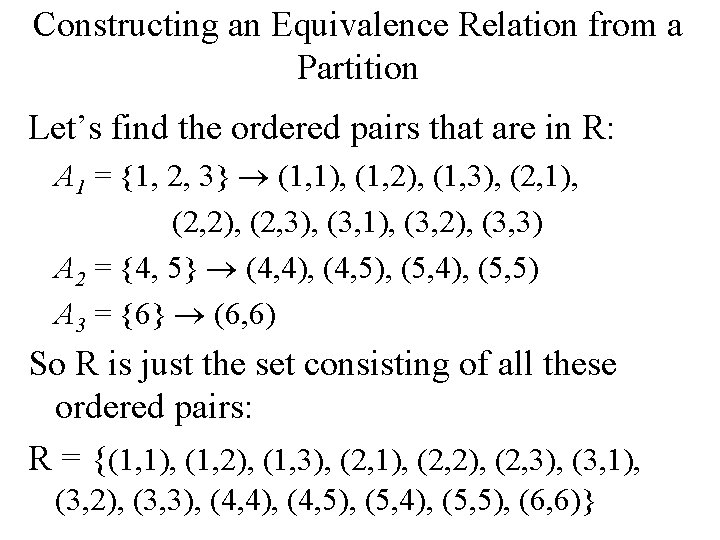Constructing an Equivalence Relation from a Partition Let’s find the ordered pairs that are in R: A 1 = {1, 2, 3} (1, 1), (1, 2), (1, 3), (2, 1), (2, 2), (2, 3), (3, 1), (3, 2), (3, 3) A 2 = {4, 5} (4, 4), (4, 5), (5, 4), (5, 5) A 3 = {6} (6, 6) So R is just the set consisting of all these ordered pairs: R = {(1, 1), (1, 2), (1, 3), (2, 1), (2, 2), (2, 3), (3, 1), (3, 2), (3, 3), (4, 4), (4, 5), (5, 4), (5, 5), (6, 6)}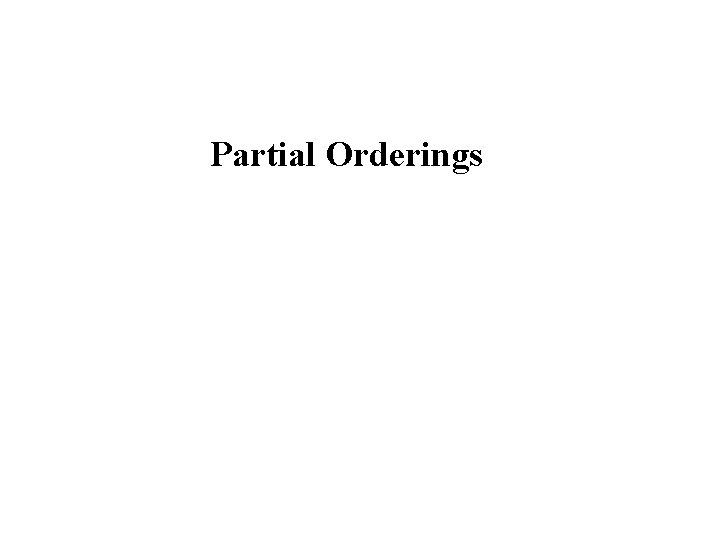Partial Orderings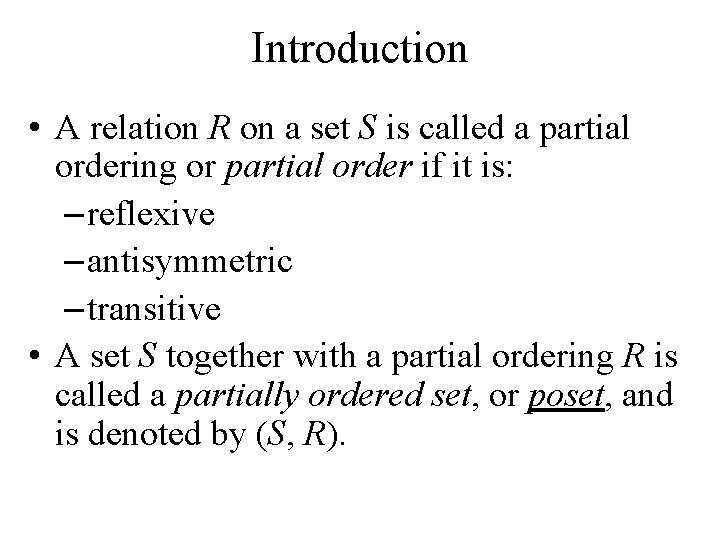Introduction • A relation R on a set S is called a partial ordering or partial order if it is: – reflexive – antisymmetric – transitive • A set S together with a partial ordering R is called a partially ordered set, or poset, and is denoted by (S, R).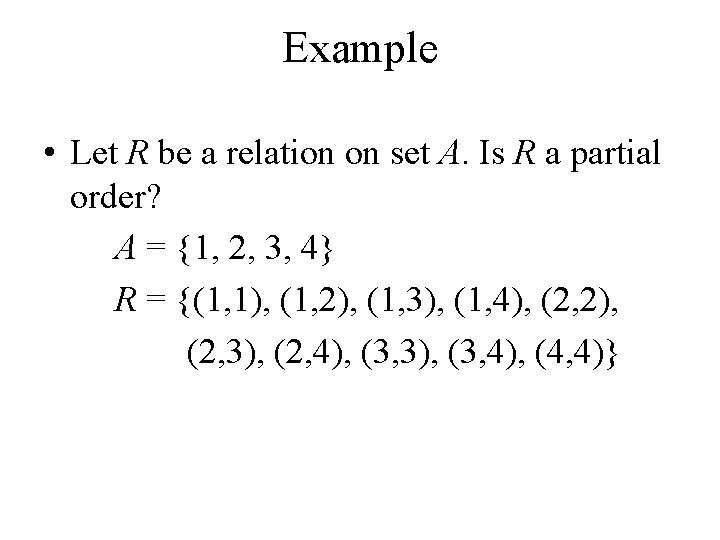Example • Let R be a relation on set A. Is R a partial order? A = {1, 2, 3, 4} R = {(1, 1), (1, 2), (1, 3), (1, 4), (2, 2), (2, 3), (2, 4), (3, 3), (3, 4), (4, 4)}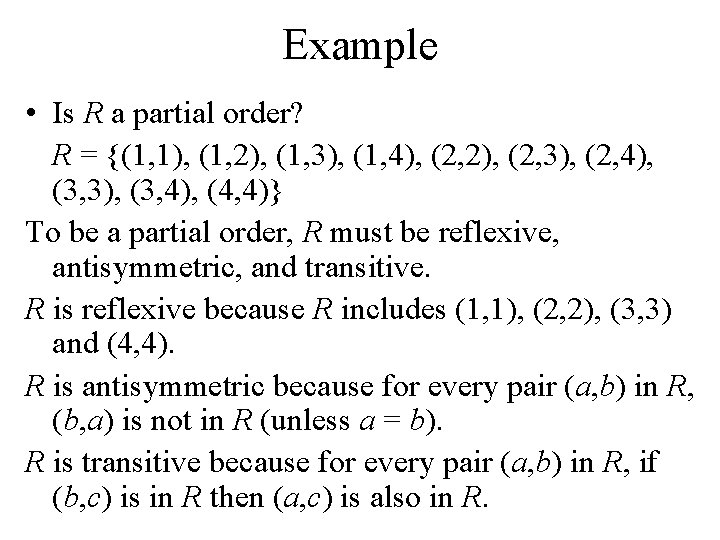Example • Is R a partial order? R = {(1, 1), (1, 2), (1, 3), (1, 4), (2, 2), (2, 3), (2, 4), (3, 3), (3, 4), (4, 4)} To be a partial order, R must be reflexive, antisymmetric, and transitive. R is reflexive because R includes (1, 1), (2, 2), (3, 3) and (4, 4). R is antisymmetric because for every pair (a, b) in R, (b, a) is not in R (unless a = b). R is transitive because for every pair (a, b) in R, if (b, c) is in R then (a, c) is also in R.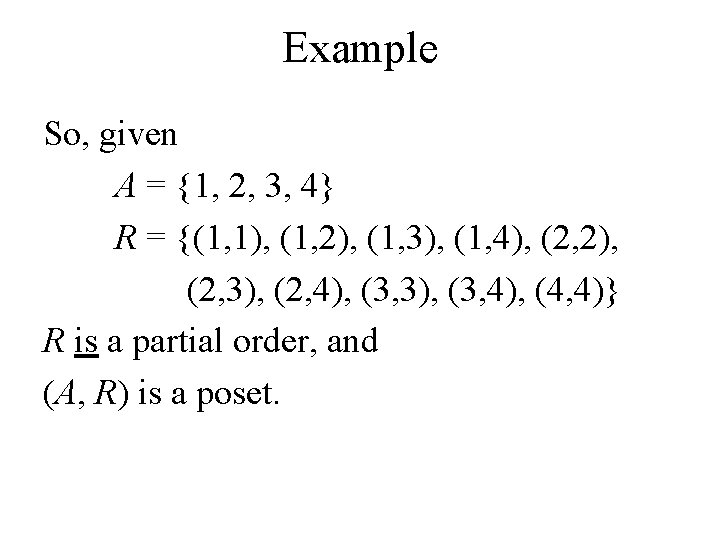Example So, given A = {1, 2, 3, 4} R = {(1, 1), (1, 2), (1, 3), (1, 4), (2, 2), (2, 3), (2, 4), (3, 3), (3, 4), (4, 4)} R is a partial order, and (A, R) is a poset.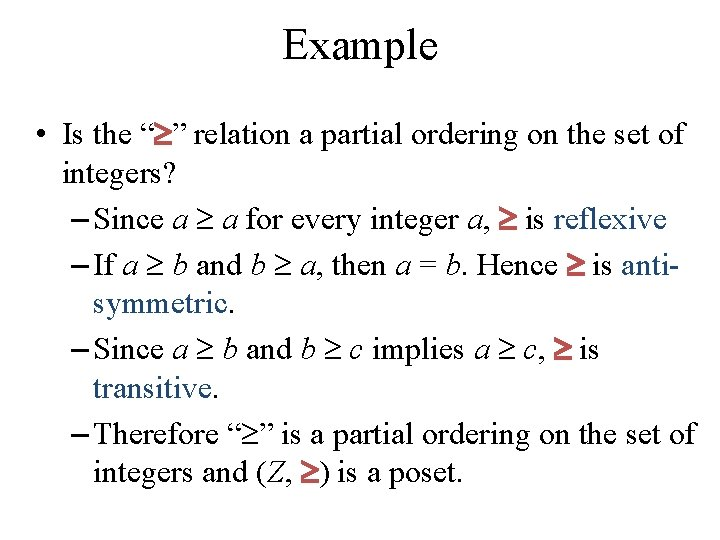Example • Is the “ ” relation a partial ordering on the set of integers? – Since a a for every integer a, is reflexive – If a b and b a, then a = b. Hence is antisymmetric. – Since a b and b c implies a c, is transitive. – Therefore “ ” is a partial ordering on the set of integers and (Z, ) is a poset.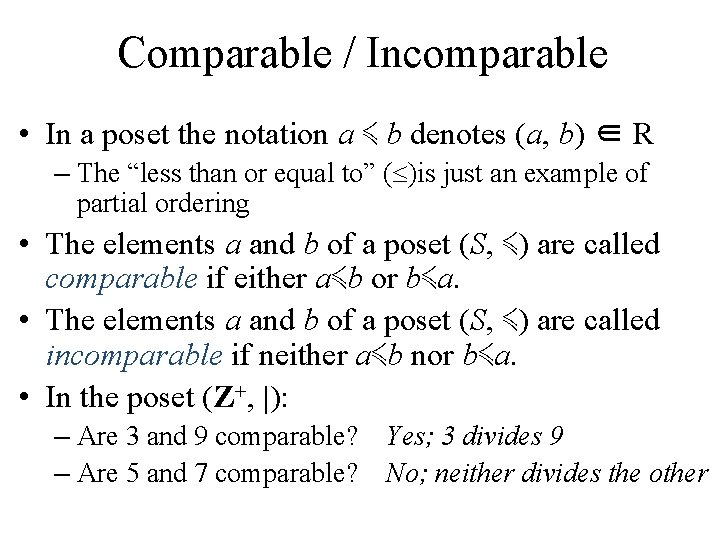Comparable / Incomparable • In a poset the notation a ≼ b denotes (a, b) ∈ R – The “less than or equal to” ( )is just an example of partial ordering • The elements a and b of a poset (S, ≼) are called comparable if either a≼b or b≼a. • The elements a and b of a poset (S, ≼) are called incomparable if neither a≼b nor b≼a. • In the poset (Z+, |): – Are 3 and 9 comparable? Yes; 3 divides 9 – Are 5 and 7 comparable? No; neither divides the otherTotal Order • We said: “Partial ordering” because pairs of elements may be incomparable. • If every two elements of a poset (S, ≼) are comparable, then S is called a totally ordered or linearly ordered set and ≼ is called a total order or linear order. • A totally ordered set is also called a chain.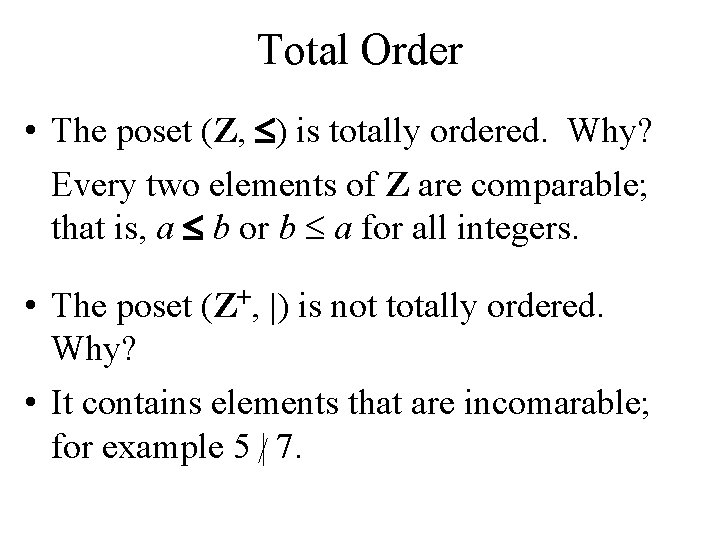Total Order • The poset (Z, ) is totally ordered. Why? Every two elements of Z are comparable; that is, a b or b a for all integers. • The poset (Z+, |) is not totally ordered. Why? • It contains elements that are incomarable; for example 5 /| 7.